Next: 3. LSVM (Lagrangian Support Up: Lagrangian Support Vector Machines Previous: 1. Introduction

# 2. The Linear Support Vector Machine

We consider the problem of classifying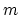points in the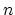-dimensional real space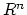, represented by the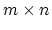matrix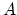, according to membership of each pointin the class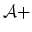or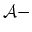as specified by a given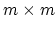diagonal matrix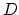with plus ones or minus ones along its diagonal. For this problem the standard support vector machine with a linear kernel [28,4] is given by the following quadratic program with parameter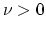: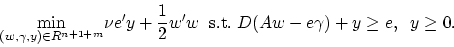(2)

Here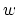is the normal to the bounding planes: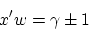(3)

and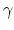determines their location relative to the origin. See Figure 1. The plane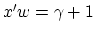bounds the classpoints, possibly with some error, and the plane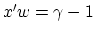bounds the classpoints, also possibly with some error. The linear separating surface is the plane: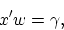(4)

midway between the bounding planes (3). The quadratic term in (2) is twice the reciprocal of the square of the 2-norm distance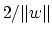between the two bounding planes of (3) (see Figure 1). This term enforces maximization of this distance, which is often called the margin''. If the classes are linearly inseparable, as depicted in Figure 1, then the two planes bound the two classes with a soft margin''. That is, they bound each set approximately with some error determined by the nonnegative error variable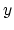: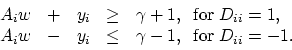(5)

Traditionally the constant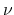in (2) multiplies the the 1-norm of the error variableand acts as a weighting factor. A nonzeroresults in an approximate separation as depicted in Figure 1.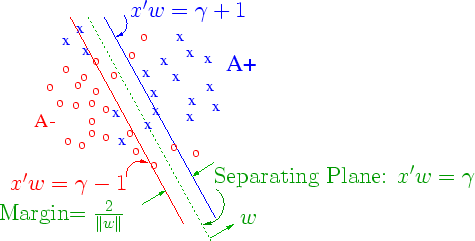The dual to the standard quadratic linear SVM (2) [15,25,16,5] is the following: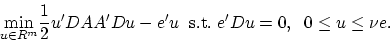(6)

The variables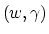of the primal problem which determine the separating surface (4) can be obtained from the solution of the dual problem above [17, Eqns. 5 and 7]. We note immediately that the matrix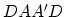appearing in the dual objective function (6) is not positive definite in general because typically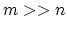. Also, there is an equality constraint present, in addition to bound constraints, which for large problems necessitates special computational procedures such as SMO  or SVM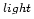. Furthermore, a one-dimensional optimization problem  must be solved in order to determine the locatorof the separating surface (4). In order to overcome all these difficulties as well as that of dealing with the necessity of having to essentially invert a very large matrix of the order of, we propose the following simple but critical modifications to the standard SVM formulation (2). We change the 1-norm ofto a 2-norm squared which makes the constraint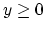redundant. We also append the term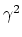to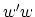. This in effect maximizes the margin between the parallel separating planes (3) by optimizing with respect to bothand, that is with respect to both orientation and location of the planes, rather that just with respect towhich merely determines the orientation of the plane. This leads to the following reformulation of the SVM: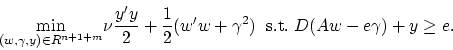(7)

The dual of this problem is :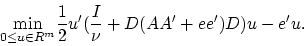(8)

The variablesof the primal problem which determine the separating surface (4) are recovered directly from the solution of the dual (8) above by the relations: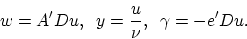(9)

We immediately note that the matrix appearing in the dual objective function is positive definite and that there is no equality constraint and no upper bound on the dual variable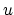. The only constraint present is a nonnegativity one. These facts lead us to our simple iterative Lagrangian SVM Algorithm which requires the inversion of a positive definite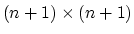matrix, at the beginning of the algorithm followed by a straightforward linearly convergent iterative scheme that requires no optimization package.Next: 3. LSVM (Lagrangian Support Up: Lagrangian Support Vector Machines Previous: 1. Introduction
Journal of Machine Learning Research